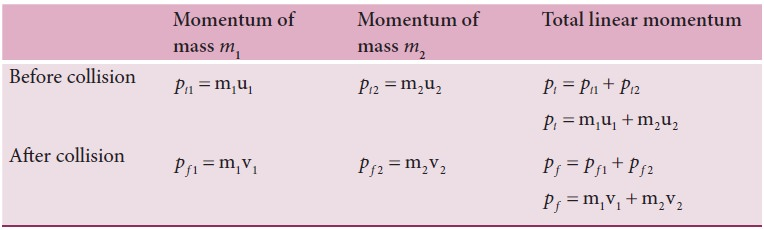Home | | Physics 11th std | Elastic collisions in one dimension

# Elastic collisions in one dimension

Consider two elastic bodies of masses m1 and m2 moving in a straight line (along positive x direction) .

Elastic collisions in one dimension

Consider two elastic bodies of masses m1 and m2 moving in a straight line (along positive x direction) on a frictionless horizontal surface as shown in figure 4.16.In order to have collision, we assume that the mass m1 moves faster than mass m2 i.e., u1 > u2. For elastic collision, the total linear momentum and kinetic energies of the two bodies before and after collision must remain the same.From the law of conservation of linear momentum,

Total momentum before collision ( pi) = Total momentum after collision ( pf )Further,For elastic collision,

Total kinetic energy before collision KEi = Total kinetic energy after collision KE fAfter simplifying and rearranging the terms,Using the formula a- b2 = (a+b)( a-b) , we can rewrite the above equation asDividing equation (4.49) by (4.47) gives,This means that for any elastic head on collision, the relative speed of the two elastic bodies after the collision has the same magnitude as before collision but in opposite direction. Further note that this result is independent of mass.

Rewriting the above equation for  vand v2,To find the final velocities v1 and v2:

Substituting equation (4.52) in equation (4.47) gives the velocity of m1 asSimilarly, by substituting (4.51) in equation (4.47) or substituting equation (4.53) in equation (4.52), we get the final velocity of m2 asCase 1: When bodies has the same mass i.e., m1 = m2,The equations (4.55) and (4.56) show that in one dimensional elastic collision, when two bodies of equal mass collide after the collision their velocities are exchanged.

Case 2:  When bodies have the same mass i.e., m1 = m2 and second body (usually called target) is at rest (u2 = 0), By substituting m1 = m2 and u2 = 0 in equations  4.53) and equations (4.54) we get,Equations (4.57) and (4.58) show that when the first body comes to rest the second body moves with the initial velocity of the first body.

Case 3:

The first body is very much lighter than the second bodythen the ratio m1/m2 ≈ 0 and also if the target is at rest ( u2=0)

Dividing numerator and denominator of equation (4.53) by m2, we getSimilarly,

Dividing numerator and denominator of equation (4.54) by m2, we getThe equation (4.59) implies that the first body which is lighter returns back (rebounds) in the opposite direction with the same initial velocity as it has a negative sign. The equation (4.60) implies that the second body which is heavier in mass continues to remain at rest even after collision. For example, if a ball is thrown at a fixed wall, the ball will bounce back from the wall with the same velocity with which it was thrown but in opposite direction.

Case 4:

The second body is very much lighter than the first bodythen the ratio m2/m1 ≈ 0 and also if the target is at rest ( u2=0)

Dividing numerator and denominator of equation (4.53) by m1, we getSimilarly,

Dividing numerator and denominator of equation (4.58) by m1, we getThe equation (4.61) implies that the first body which is heavier continues to move with the same initial velocity. The equation (4.62) suggests that the second body which is lighter will move with twice the initial velocity of the first body. It means that the lighter body is thrown away from the point of collision.

## Solved Example Problems for Elastic collisions in one dimension

### Example 4.20

A lighter particle moving with a speed of 10 m s-1 collides with an object of double its mass moving in the same direction with half its speed. Assume that the collision is a one dimensional elastic collision. What will be the speed of both particles after the collision?

### SolutionLet the mass of the fi rst body be m which  moves with an initial velocity, u1 = 10 m s-1.

Therefore, the mass of second body is 2m and its initial velocity is u2 = ½ u1 = ½(10ms-1)

Then, the fi nal velocities of the bodies can be calculated from the equation (4.53) and equation (4.54)As the two speeds v1and v2 are positive, they move in the same direction with the velocities, 3.33 m s−1 and 8.33 m s−1 respectively.

Study Material, Lecturing Notes, Assignment, Reference, Wiki description explanation, brief detail
11th Physics : UNIT 4 : Work, Energy and Power : Elastic collisions in one dimension |